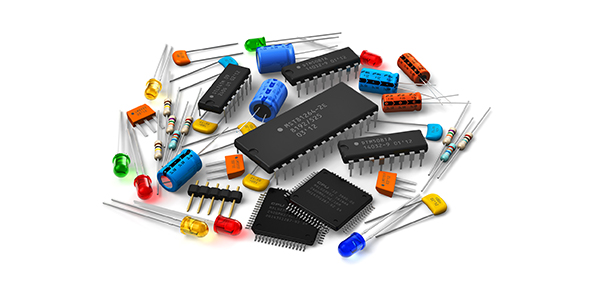# Power Electronics Trivia Questions And Answers

20 Questions | Attempts: 991
ShareSettingsCharacteristics of Power Electronic devices, phase control, bridge converters, choppers and inverters, basic concepts of adjustable speed drives.

• 1.
Triacs cannot be used in a.c voltage regulator for a
• A.

• B.

• C.

• D.

• 2.
In a step-down cyclo converter commutation required is
• A.

Natural

• B.

Forced

• C.

Depends on power

• D.

None of these

• 3.
Cyclo converter can be considered as a.c-a.c converter with conversion of
• A.

1 stage

• B.

2 stage

• C.

3 stage

• D.

None of these

• 4.
Inverters are used in
• A.

Induction heating

• B.

UPS

• C.

None of these

• D.

Both

• 5.
Commutation overlap in the phase controlled ac to dc converter is due to
• A.

• B.

• C.

Switching operator in the converter

• D.

Source inductance

• 6.
Which of the following devices should be used as a switch in a low power switched mode power supply(SMPS)
• A.

GTO

• B.

MOSFET

• C.

TRIAC

• D.

THYRISTOR

• 7.
A converter which can operate in both 3-phase and 6-pulse modes is a
• A.

1-phase full converter

• B.

3-phase half wave converter

• C.

3-phase semi converter

• D.

3-phase full converter

• 8.
In d.c choppers per unit ripple is maximum when duty cycle alpha is
• A.

0.1

• B.

0.3

• C.

0.5

• D.

0.7

• 9.
The most suitable device for high frequency inversion in SMPS is
• A.

BJT

• B.

IGBT

• C.

MOSFET

• D.

GTO

• 10.
Which of the following doesnot cause permanent damage of an SCR
• A.

High current

• B.

High rate of rise of current

• C.

High temperature rise

• D.

High rate of raise of voltage

• 11.
A 4-quadrant chopper cannot be operated as
• A.

• B.

Cyclo converter

• C.

Inverter

• D.

Bi-directional rectifier

• 12.
Power diodes has characteristics of
• A.

High power,high speed

• B.

Low power,low speed

• C.

Low power,high speed

• D.

High power,low speed

• 13.
IGBT is use for applications in
• A.

Low power

• B.

Medium power

• C.

High power

• D.

None of these

• 14.
Turn-on and Turn-off times of transistor depend on
• A.

Static characteristics

• B.

Junction capacitance

• C.

Current gain

• D.

None of these

• 15.
Which one of the following is used as the main switching element in a switched mode power supply operating in 20khz to 100khz range?
• A.

Thyristor

• B.

MOSFET

• C.

TRIAC

• D.

UJT

• 16.
When an UJT is used for triggering an SCR, the waveshape of the voltage obtained from UJT  circuit is a
• A.

Sine wave

• B.

Saw-tooth wave

• C.

Trapezoidal wave

• D.

Square wave

• 17.
In a 3-phase semi-converter, for firing angle less than or equal to 60degrees, free wheeling diode conducts for
• A.

30 degrees

• B.

60 degrees

• C.

90 degrees

• D.

0 degrees

• 18.
In a 1-phase full converter, if load current is I and ripple free, than average thyristor current is
• A.

1/2*I

• B.

1/3*I

• C.

1/4*I

• D.

I

• 19.
Power electronic device with poor turn-off gain is
• A.

A symmetrical thyristor

• B.

A conventioanal thyristor

• C.

Power bipolar junction transistor

• D.

Gate turn-off thyristor

• 20.
Modern ac-dc converters employ self commutated devices like GTOs of IGBTs instead of thyristors inorder to achieve
• A.

• B.

Better efficiency in conversion

• C.

Low switching frequency operation of devices

• D.

Cost reduction in converter realization

## Related TopicsBack to top
×

Wait!
Here's an interesting quiz for you.